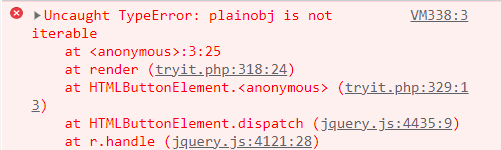Open in App
Not now

# What are these triple dots (…) in JavaScript ?

• Last Updated : 23 Feb, 2023

In JavaScript, there are multiple ways in which we can assign the value of one object to another. Sometimes we do not know the exact number of arguments that are to be assigned. In this case, the triple dots are used.

The triple dots are known as the spread operator, which allows iterable values to be assigned as it gives a list of parameters to the object. The spread operator is useful as it reduces the amount of code to be written and increases its readability. The syntax of the spread operator is the same as the rest parameter but the working is different. It was introduced as a feature in ES6 JavaScript

Syntax:

`var nameOfVar = [...valueToSpread];`

Parameter:

• valueToSpread: The iterable that is to be assigned to the new variable is mentioned here. It can be an Array or a String.

Return Type: It returns the argument list of the object passed after three dots.

Let us see some examples where we assign value to objects first by a simple method, then we use triple dots or spread syntax to perform the same operations.

Example 1: In this example, we will concatenate two arrays using the inbuilt concat method and then perform the same task using triple dots syntax.

## Javascript

 `var` `baseArr = [1, 2, 3];` `var` `baseArr2 = [4, 5, 6];`   `// Inbuilt concat method` `baseArr = baseArr.concat(baseArr2); ` `console.log(baseArr);`   `var` `spreadArr = [``'a'``, ``'b'``, ``'c'``];` `var` `spreadArr2 = [``'d'``, ``'e'``, ``'f'``];`   `// Concatenating using three dots` `spreadArr = [...spreadArr, ...spreadArr2]; ` `console.log(spreadArr);`

Output:

```[1, 2, 3, 4, 5, 6]
['a', 'b', 'c', 'd', 'e', 'f']```

Example 2: In this example, we will copy the contents of an array with the assignment operator and the spread operator:

## Javascript

 `var` `baseArr = [1, 2, 3];` `var` `baseArr2 = baseArr;` `baseArr2.push(4);` `console.log(baseArr2);` `console.log(baseArr);`   `var` `spreadArr = [``'a'``, ``'b'``, ``'c'``];` `var` `spreadArr2 = [...spreadArr];` `spreadArr2.push(``'d'``);` `console.log(spreadArr);` `console.log(spreadArr2);`

Output:

```[1, 2, 3, 4]
[1, 2, 3, 4]
['a', 'b', 'c']
['a', 'b', 'c', 'd']```

Explanation: We can see that when assigning the array using the assignment operator(‘=’) and modifying the new array, the old array is also modified. This causes a problem as we do not always want to modify the content of the old array but if we use spread operator syntax to assign values and modify the new array, the old array is left unchanged. This happens because a new array is returned by the spread operator instead of the reference of the old array.

Example 3: In this example, we will find the min of an array using the Math.min() method:

## Javascript

 `var` `baseArr = [5, 2, 7, 8, 4, 9];` `console.log(Math.min(baseArr));` `console.log(Math.min(...baseArr));`

Output:

```NaN
2```

Explanation: Math.min() method requires an object list to find the minimum inside the list but when passing an array it gives NaN output. To overcome this problem we used the triple dots format/spread operator. As the spread operator returns a list of objects it is accepted by the method and the minimum element of the array is returned.

Example 4: In this example, we will assign an object to another object using the three dots:

## Javascript

 `const spreadObj = {` `    ``name: ``'Ram'``,` `    ``country: ``'India'``,` `};`   `// Cloning previous object` `const newObj = { ...spreadObj }; ` `console.log(newObj);`

Output:

`{name: 'Ram', country: 'India'}`

Example 5: In this example, we will use the spread operator in a function call

## Javascript

 `function` `add(...objects){` `    ``var` `ans = 0;` `    ``for``(``var` `i = 0; i < objects.length; i++){` `        ``ans += objects[i];` `    ``}` `    ``console.log(ans);` `}` `add(1, 2);` `add(23, 45, 67, 56);`

Output:

```3
191```

Explanation: We can see that the spread operator is useful when we do not know the number of arguments that are to be passed in a function. Here our add function works for any number of arguments so we do not have to specify multiple functions for a different number of arguments.

NOTE: We should keep in mind that only those objects can be passed using the spread operator which is iterable. For eg., plain objects are not iterable as they lack the Symbol.iterator method.

Example 6: In this example, we will try spreading a plain object.

## Javascript

 `const plainObj = { name: ``'Ram'` `}; ``// Spreading non iterable object` `const baseArr = [...plainobj];`

Output:Explanation: We can see that when trying to spread a non-iterable object using the three dots an error is thrown.

My Personal Notes arrow_drop_up
Related Articles